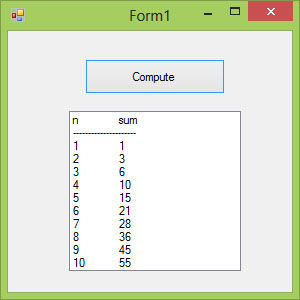# Visual Basic 2013 Lesson 15: Looping

#### [Lesson 14] << [Contents] >> [Lesson 16]

A procedure in a computer program that runs repeatedly until meeting the certain condition is called looping. A loop can go on repetitively as long as the processor and memory could support.  For example, a program that adds a series of numbers until the sum exceeds a certain value or a program that prompts the user to enter data repeatedly until he or she enters the word ‘Finish’.

In Visual Basic 2013, there are three methods of Looping,  the For…..Next loop, the Do loop, and the While…..End While loop. All methods produce the same repetitive effects.

### 15.1 Looping using For…Next Loop

The most common looping method in VB 2013 is the For….Next loop. The structure of a For…Next loop is as shown below:

```For counter=startNumber to endNumber Step increment
One or more statements
Next
```

To exit a For…..Next Loop, you can place the Exit For statement within the loop; it is normally used together with the If….Then statement. For its application, you can refer to example 15.1 d.

Example 15.1 a

```Dim counter as Integer
For counter=1 to 10
Next
```

* The program will enter number 1 to 10 into the list box.

#### Example 15.1b

```Dim counter , sum As Integer
For counter=1 to 100 step 10
sum+=counter
Next
```

* The programme will calculate the sum of the numbers as follows:

sum=0+10+20+30+40+……

#### Example 15.1c

```Dim counter, sum As Integer
sum = 1000
For counter = 100 To 5 Step -5
sum – = counter
Next
```

*Notice that increment can be negative.

The program will compute the
subtraction as follow:
1000-100-95-90-……….

#### Example 15.1d

```Dim n as Integer
For n=1 to 10
If n>6 then
Exit For
End If
Else
Next
End If
Next
```

The process will stop when n is greater than 6.

### 15.2 Looping using Do Loop

The Do Loop structures are

a)

```Do While condition
Block of one or more statements
Loop
```

b)

```Do
Block of one or more statements
Loop While condition
```

c)

```Do Until condition
Block of one or more statements
Loop
```

d)

```Do
Block of one or more statements
Loop Until condition
```

Sometimes we need exit to exit a loop prematurely because a certain
condition is fulfilled. The syntax to use is Exit Do. Let’s examine the following examples:

#### Example 15.2(a)

```Do while counter <=1000
TextBox1.Text=counter
counter +=1
Loop
```

* The above example will keep on adding until counter >1000.

The above example can be rewritten as

```Do
TextBox1.Text=counter
counter+=1
Loop until counter>1000
```

#### Example 15.2(b)

```Private Sub Button1_Click(ByVal sender As System.Object, ByVal e As System.EventArgs) Handles Button1.Click
Dim sum, n As Integer
Do
n += 1
sum += n
If n = 100 Then
Exit Do
End If
Loop
End Sub
```

* The loop in the above example can be replaced by the following loop:

```Do Until n = 10
n += 1
sum += n
Loop
```

The output is as shown in Figure 15.1Figure 15.1

### 15.3 Looping using While….End While Loop

The structure of a While….End While Loop is very similar to the Do Loop. it takes the following form:

```While conditions

Visual Basic 2013 statements

End While
```

Example 15.3

```Private Sub Button1_Click(sender As Object, e As EventArgs) Handles Button1.Click
Dim sum, n As Integer